﻿ 串联离心泵过流部件空化及磨损的数值模拟

# 串联离心泵过流部件空化及磨损的数值模拟Numerical Simulation of Cavitation and Wear of Flow Passage Parts in Series Centrifugal Pumps

Abstract: In order to analyze the flow of sand-containing water through the centrifugal pump, the damage of the flow-through components is due to the influence of sediment abrasion or cavitation; and a series centrifugal pump model is designed. Based on CFturbo software to model the centrifugal pump in series, and optimized by UG software, using ICEM for meshing. Finally, the numerical simulation of the internal flow field of a centrifugal pump in series with sandy water medium was performed by CFX software. The results show: Cavitation damage to the centrifugal pump can be reduced by in-creasing the inlet pressure of the sandy water medium into the centrifugal pump. It does not change the position where the centrifugal pump’s flow passage components are worn out. Centrifugal pump cavitation damage is mainly concentrated in the impeller inlet. The inlet of the suction surface of the blade and the outlet of the pressure surface are locations where the centrifugal pump is mainly exposed to sediment.

1. 引言

2. 计算区域及设置

2.1. 离心泵的基本设计参数

2.2. 离心泵三维建模及网格划分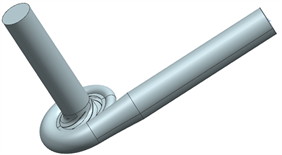Figure 1. Three-dimensional model of the water body of the centrifugal pump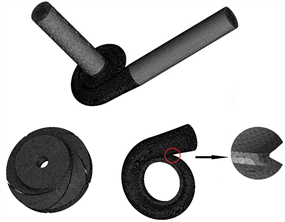Figure 2. Mesh division of water body of centrifugal pump

2.3. 边界条件设置

1) 进口边界条件：计算进口边界处，以进口总压作为进口条件，并假定进口压力垂直于进口表面，且进口处含沙水颗粒体积分数均匀分布。

2) 出口边界条件：以质量流量速率作为出口条件。

3) 固壁边界条件：过流部件的壁面边界设置为无穿透、无滑移边界条件。

2.4. 相的设置

3. 计算模型基本方程

$\frac{\partial {\varnothing }_{f}}{\partial \tau }+\frac{\partial \left({\varnothing }_{f}{V}_{fi}\right)}{\partial {x}_{i}}=0$ (3.1)

$\frac{\partial {\varnothing }_{p}\text{​}}{\partial \tau }+\frac{\partial \left({\varnothing }_{p}{V}_{pi}\right)}{\partial {x}_{i}}=0$ (3.2)

$\begin{array}{l}\frac{\partial }{\partial t}\left({\varnothing }_{f}{V}_{fi}\right)+\frac{\partial }{\partial {x}_{k}}\left({\varnothing }_{f}{V}_{fi}{V}_{fK}\right)\\ =-\frac{1}{{\rho }_{f}}{\varnothing }_{f}\frac{{\partial }_{p}}{\partial {x}_{i}}+{\theta }_{f}\frac{\partial }{\partial {x}_{k}}\left[{\varnothing }_{f}\left(\frac{\partial {V}_{fi}}{\partial {x}_{k}}+\frac{\partial {V}_{fk}}{\partial {x}_{i}}\right)\right]-\frac{B}{{\rho }_{f}}{\varnothing }_{f}{\varnothing }_{p}\left({V}_{fi}-{V}_{pi}\right)+{\varnothing }_{fgi}\end{array}$ (3.3)

$\begin{array}{l}\frac{\partial }{\partial t}\left({\varnothing }_{f}{V}_{fj}\right)+\frac{\partial }{\partial {x}_{k}}\left({\varnothing }_{f}{V}_{fj}{V}_{fK}\right)\\ =-\frac{1}{{\rho }_{f}}{\varnothing }_{f}\frac{{\partial }_{p}}{\partial {x}_{j}}+{\theta }_{f}\frac{\partial }{\partial {x}_{k}}\left[{\varnothing }_{f}\left(\frac{\partial {V}_{fj}}{\partial {x}_{k}}+\frac{\partial {V}_{fk}}{\partial {x}_{j}}\right)\right]-\frac{B}{{\rho }_{f}}{\varnothing }_{f}{\varnothing }_{p}\left({V}_{fj}-{V}_{pj}\right)+{\varnothing }_{fgj}\end{array}$ (3.4)

$\begin{array}{l}\frac{\partial }{\partial t}\left({\varnothing }_{p}{V}_{pi}\right)+\frac{\partial }{\partial {x}_{k}}\left({\varnothing }_{p}{V}_{pi}{V}_{pK}\right)\\ =-\frac{1}{{\rho }_{p}}{\varnothing }_{p}\frac{{\partial }_{p}}{\partial {x}_{i}}+{\theta }_{p}\frac{\partial }{\partial {x}_{k}}\left[{\varnothing }_{p}\left(\frac{\partial {V}_{pi}}{\partial {x}_{k}}+\frac{\partial {V}_{pk}}{\partial {x}_{i}}\right)\right]-\frac{B}{{\rho }_{p}}{\varnothing }_{f}{\varnothing }_{p}\left({V}_{fj}-{V}_{fi}\right)+{\varnothing }_{pgj}\end{array}$ (3.5)

4. 模拟结果及分析

4.1. 串联离心泵过流部件压力分析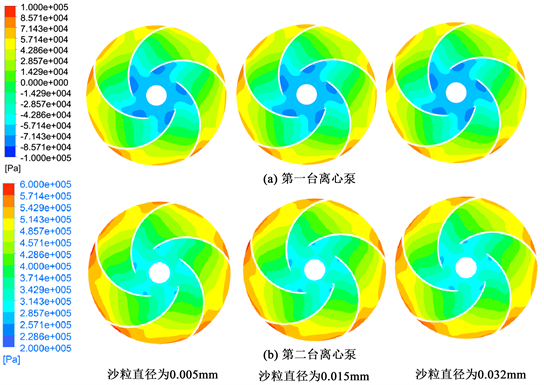Figure 3. Pressure distribution of the back cover of the tandem centrifugal pump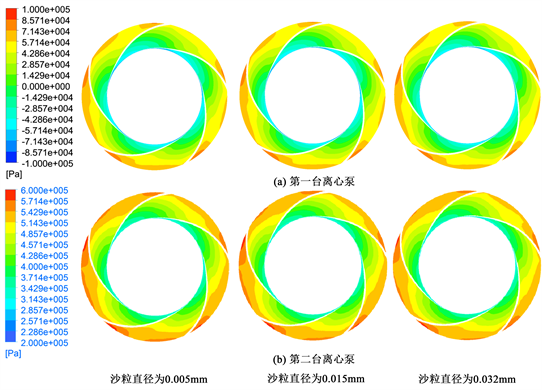Figure 4. Pressure distribution of the front cover of the tandem centrifugal pump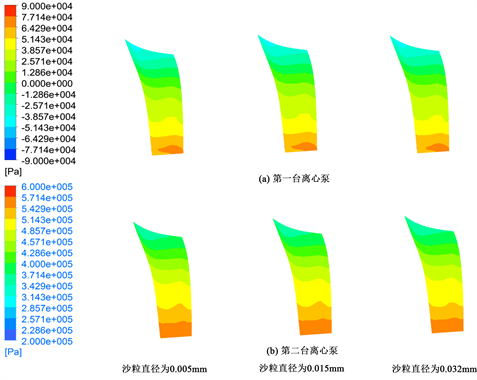Figure 5. Pressure distribution of pressure surface of series centrifugal pump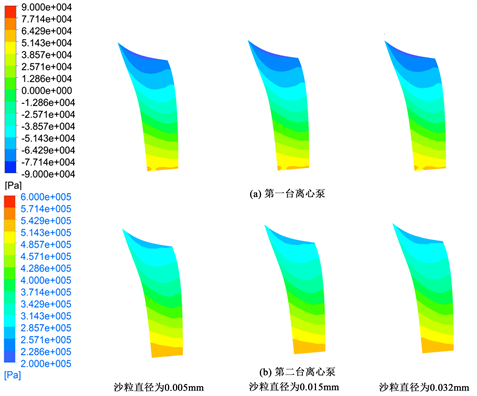Figure 6. Pressure distribution on the suction surface of a tandem centrifugal pump

4.2. 串联离心泵过流部件磨损分析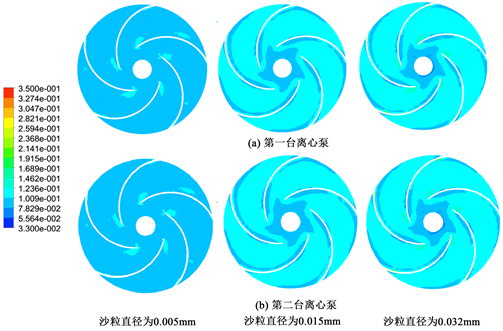Figure 7. Distribution of sand particles in the back cover of the tandem centrifugal pump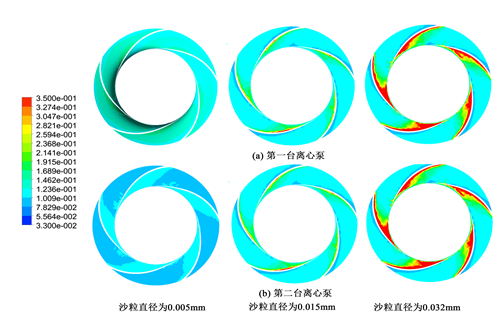Figure 8. Sand distribution in the front cover of the tandem centrifugal pump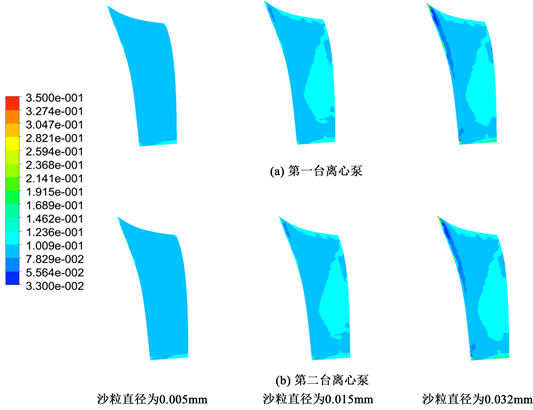Figure 9. Distribution of sand particles on pressure surface of tandem centrifugal pump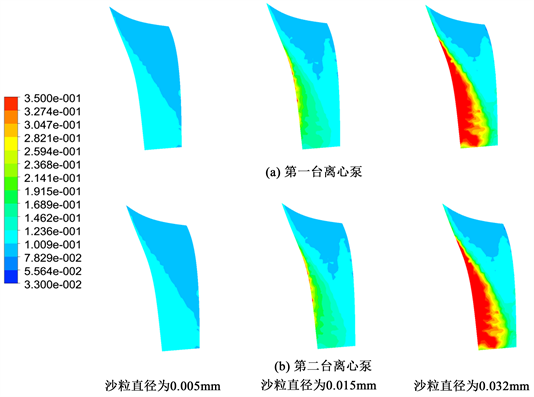Figure 10. Distribution of sand particles on the suction surface of a tandem centrifugal pump

5. 结论

NOTES

*通讯作者。

 杜同. 含砂水中泵损坏原因新解[J]. 水泵技术, 199(1): 3-9.

 杜同. 含砂水中汽蚀和磨损规律的研究[J]. 机械, 1980(11): 1-8+68.

 杜同. 含砂水中水力机械的汽蚀破坏和泥砂磨损规律的研究[J]. 机械工程材料, 1978(5): 1-11.

 杜同. 消灭汽蚀是彻底解决含砂水中水轮机、水泵快速破坏的正确方向——含砂水中汽蚀和磨损规律的研究[J]. 大自然探索, 1984(1): 113-121.

 魏立超, 宋文武, 石建伟, 等. 孔板前后倾角对高速离心泵流场特性的影响[J]. 机械科学与技术, 2017, 36(8): 1180-1186.

 胡帅, 宋文武, 庞辉, 魏立超. 微型高速泵小流量工况空化特性数值分析[J]. 热能动力工程, 2017, 32(8): 100-106+151.

 庞辉, 宋文武, 胡帅. 含砂水对混流泵过流部件磨损的数值模拟[J]. 热能动力工程, 2017, 32(12): 90-95+141.

 赵琴. 浑水异重流两相流模型的比较研究[J]. 西华大学学报(自然科学版), 2012, 31(3): 100-104.

Top